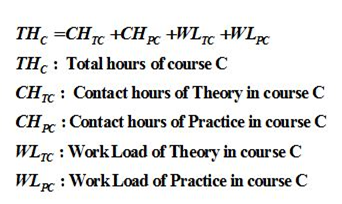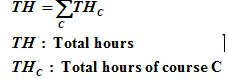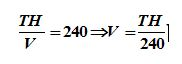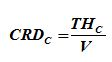# Credit hours and calculating details for each course

Credit hours and calculating details for each course

The system of credit hours adopted in the Syrian Virtual University programs has been made to conform to the standards and regulations of the Ministry of Higher Education and international standards which will facilitate any scientific cooperation agreement with any international academic body in the future; and in accordance with Decree No. 245 of 2010, concerning the amendment of the Executive Regulations of the Law on Organizing Universities No. 250 of 2006, and founder of the system of credit hours.

The number of approved annual credit hours will be between 48 and 72 in accordance with Decree 245 and at the same time to meet the Bologna standards of the European Union.

1. Calculation of credit hours according to the European system:

The calculation of credit hours according to the European system depends on the number of teaching hours (Contact hours CH) and the number of hours spent by student in studying and the preparing of Work Load (WL), which indicates the time spent by the student to complete all educational activities such as lectures, projects, Self-learning, and exams.

To facilitate the calculation of work load WL, we take into account that every 1 hour of theoretical teaching requires a personal effort by the student ranging from a minimum of (1) hour to a maximum of (2) hours, and every 1 hour of practical teaching requires a personal effort by the student ranging from a minimum of (0) Hour to a maximum of 1 hour of personal effort. At the end of the day, each value of 20 to 30 hours of work load is considered an ECTS approved European credit hour. To implement the European Credit Hour Account we do the following:

1. The total number of hours per course is calculated according to the following:2. Total hours of courses are calculated per year:The TH value should be limited to 1200 hours of effort and 1800 hours.

3. The value of the coefficient V (which we will use in calculating the number of credit hours of a course considering the number of effort hours in the course) by dividing the total number of hours obtained by studying all the courses required to obtain the ETCS 240 (The number of required credit hours to obtain a university degree according to the European system):It should be noted here that it is possible not to calculate the value of factor V and to agree on using a fixed value between 25 and 30, where the value of 25 is the value adopted by the university.

4. We then go back to each course and divide the total number of hours of the course on the previous value to get the number of credit hours:2. Rules of distribution of contact hours and hours of effort and calculating courses’ credit hours:

In accordance with the teaching rules and its mechanisms at the Syrian Virtual University, the definition of contact hours is as follows:

1. The recorded hour accompanying the courses is considered as a theoretical contact hour.

2. The synchronized hour fulfilled by the teacher at the Syrian Virtual University is a practical hour. The number of these hours should preferably be 18 hours per course. This will ensure that 12 synchronized sessions-one hour and a half each per lecture or class- are achieved and all in accordance with the lecture systems and all associated regulations at the University.

3. Application Example:

Suppose that we have a course. The number of hours of lectures recorded is 18 hours, and the amount of effort required to study them is similar that is 18 hours. Suppose that the number of hours of synchronized sessions is 18 hours, and that the amount of effort required to understand them is equal to 18, (18 + 18 + 18 + 18) The number of credit hours for this course is calculated according to the European system (rounded to the nearest integer): CRD = 18 + 18 + 18 + 18 ) / 25 = 3